Open In App
Related Articles
• Geometry in Maths

# Geometry in Maths

Geometry is one of the oldest branches of mathematics that is concerned with the shape, size, angles, and dimensions of objects in our day-to-day life.

Geometry in maths plays a crucial role in understanding the physical world around us and has a wide range of applications in various fields, from architecture and engineering to art and physics. There are two types of shapes in Euclidean Geometry: Two dimensional and Three-dimensional shapes. Flat shapes are 2D shapes in plane geometry that include triangles, squares, rectangles, and circles. 3D shapes in solid geometry such as a cube, cuboids, cones, and so on are also known as solids. Fundamental geometry is based on points, lines, and planes, as described in coordinate geometry.

In this article, you will learn everything related to Geometry like what is geometry, branches of geometry, different types of geometry, its examples, etc.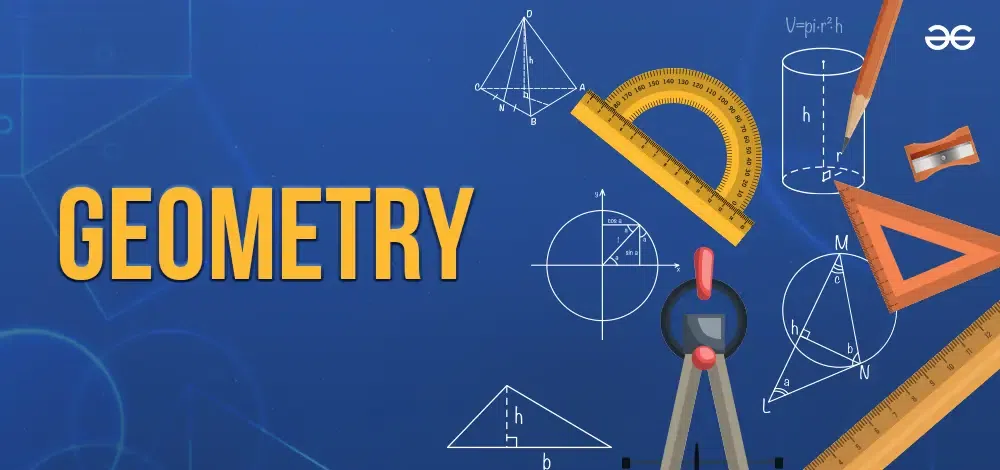Table of Content

## What is Geometry in Maths?

The word Geometry is made up of two Ancient Greek words- ‘Geo’ means ‘Earth’ and ‘metron’ means ‘measurement’. Geometry is the study of different varieties of shapes, figures, and sizes. It gives us knowledge about distances, angles, patterns, areas, and volumes of shapes. The principles of geometry depend on points, lines, angles, and planes. All the geometrical shapes are based on these geometrical concepts.

### Geometry Definition

Geometry is the unit of mathematics in which we study different types of shapes, figures and sizes in Maths or in real life.

## Branches of Geometry

The geometry can be divided into different parts:

• Algebraic Geometry
• Discrete Geometry
• Differential Geometry
• Euclidean Geometry
• Non-Euclidean Geometry(Elliptical Geometry and Hyperbolic Geometry)
• Convex Geometry
• Topology

### Algebraic Geometry

This branch of geometry focuses on the zeros of the multivariate polynomial. It consists of linear and polynomial algebraic equations for solving sets of zeros. Applications in this category include string theory and cryptography.

### Discrete Geometry

This branch of geometry mainly focuses on the position of simple geometrical objects such as points, lines, triangles, etc. It includes problems based on ordinary continuous spaces that have a combinatorial aspect.

### Differential Geometry

It comprises algebraic and calculus techniques for problem-solving. The various problems include problems like general relativity in physics etc.

### Euclidean Geometry

In Euclidean geometry, we study planes and solid figures based on axioms and theorems. The fundamental theorems of Euclidean geometry include Points and Lines, Euclid’s Axioms and Postulates, Geometrical Proof, and Euclid’s Fifth Postulate. It has multiple applications in the fields of Computer Science, Mathematics, etc.

The five postulates of Euclidean geometry are as follows:

• A straight line can be drawn from one given point to another.
• The length of a straight line is infinite in both directions.
• Any specified point can serve as the circle’s center and any length can serve as the radius.
• All right angles are congruent.
• Any two straight lines that are equal in distance from one another at two points are infinitely parallel.

Some of Euclid’s axioms in geometry that are universally accepted are :

• The things that are equal to the same things are equal. If A = C and B = C then A = C
• If equals are added to equals, the wholes are equal. If A = B and C = D, then A + C = B + D
• If equals are subtracted, the remainders are equal.
• The coinciding things are equal t
• The whole is greater than its part. If A > B, then there exists C such that A = B + C.
• The things that are double the same are equal.
• The things that are halves of the same thing are equal

### Non-Euclidean Geometry

There are two types of Non-Euclidean Geometry- Spherical and Hyperbolic Geometry. It is different from Euclidean geometry due to the difference in the principles of angles and parallel lines.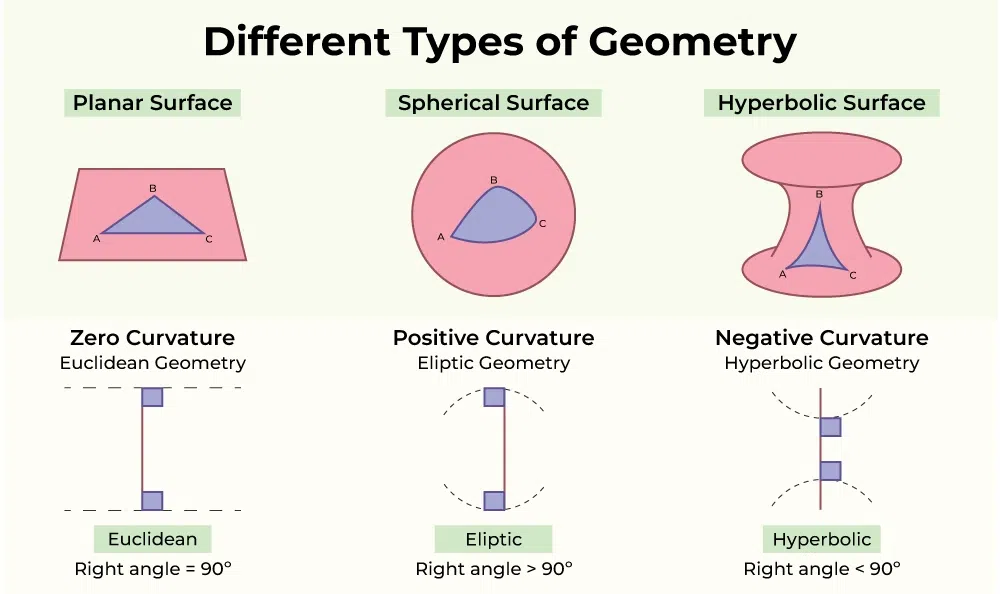Non-Euclidean Geometry

The study of plane geometry on the sphere is known as spherical geometry. The sum of angles in the triangle is greater than 180°.
A curved surface is referred to as hyperbolic geometry. Topology is where this geometry is used. The planar triangle has a total of angles that is less than 180 degrees, depending on the interior curvature of the curved surface.

### Convex Geometry

It consists of convex shapes in Euclidean space and uses techniques that involve real analysis. It is used in various applications of optimization and functional analysis.

### Topology

It comprises the properties of space that are under continuous mapping. It is used in consideration of compactness, completeness, continuity, filters, function spaces, grills, clusters and bunches, hyperspace topologies, initial and final structures, metric spaces, nets, proximal continuity, proximity spaces, separation axioms, and uniform spaces.

## Plane Geometry

Plane geometry is concerned with the shapes that can be drawn on paper. Euclidean geometry involves the study of plane geometry. A 2D surface spread infinitely in both directions is referred to as a plane. The basic components of the plane are:

• Points
• Lines
• Angles

A point is the no-dimensional fundamental unit of geometry. Collinear points are the ones that lie on the same line. A line is a straight path on a plane that extends in both directions with no endpoints. A line segment is part of a line that has two endpoints and is finite in length. A ray is a line segment that extends indefinitely in one direction. A line has no endpoints. Line, line segment, and ray are different from each other. Plane geometry consists of lines, circles, and triangles of two dimensions. Plane geometry is another name for two-dimensional geometry.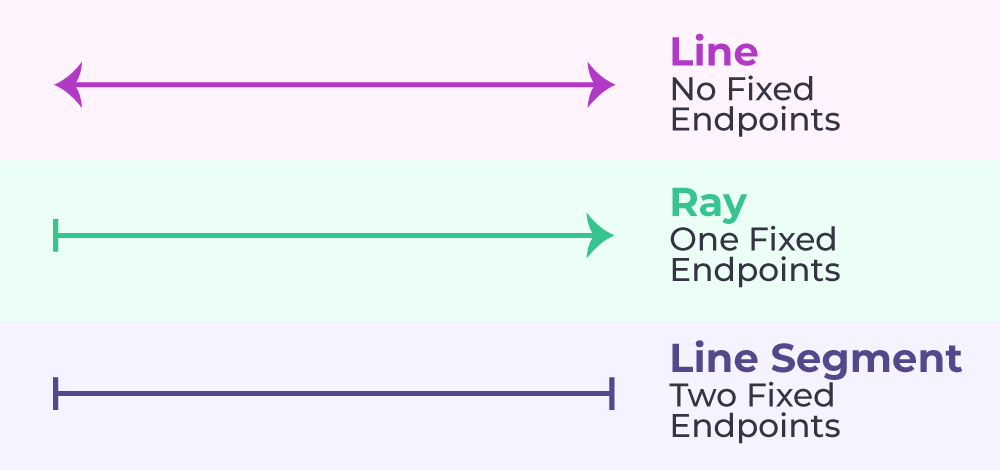Plane Geometry

All two-dimensional figures have only two dimensions: length and width. Plane figures consist of squares, triangles, rectangles, circles, and so on.

### Angles in Geometry

In planar geometry, an angle is formed when two rays intersect, called the sides of the angle, and share a common endpoint known as the vertex of the angle.

There are majorly four types of angles

Acute Angle-  An Angle between 0 to 90 degrees.

Obtuse Angle– An angle more than 90 degrees but less than 180 degrees.

Right Angle– An angle of 90 degrees.

Straight Angle– An angle of 180 degrees is a straight line.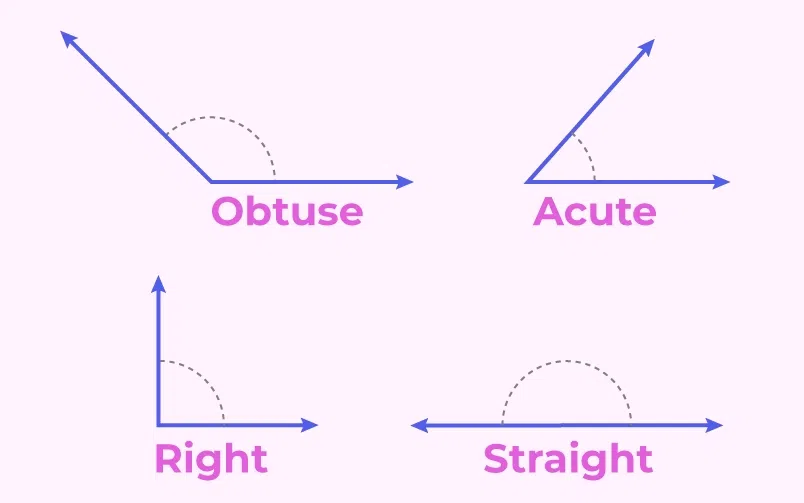Angles in Geometry

You can find similar topics discussed in depth in the below articles.

### Polygon and its types

A figure that is made up of a finite number of straight-line segments closing in a loop. The word ‘poly’ means multiple.

The sum of internal angles of a polygon is : (n-2) * 180

where n is the number of sides.

### Types of Polygon in Geometry

The types of polygons are:

• Triangles
• Pentagon
• Hexagon
• Heptagon
• Octagon
• Nonagon
• Decagon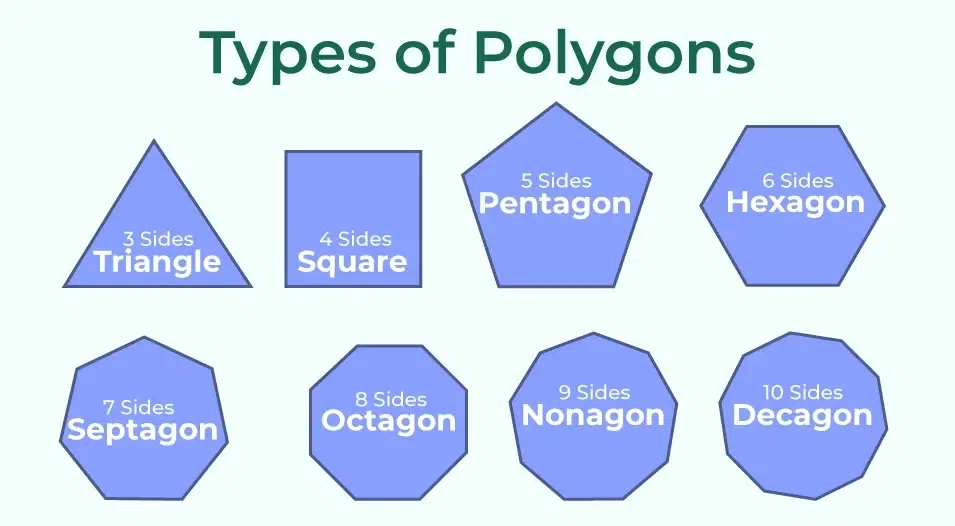Types of Polygon

Here is a list of articles where you can find in-depth knowledge about the above topic.

### Circle in Geometry

A circle is a closed shape. From a fixed point known as the center, all the points of a circle are of the same distance.Here is a list of articles where you can find in-depth knowledge about circles.

### Similarity and Congruency in Geometry

Similarity: Two figures are considered similar if they have the same shape or equal angle but may not be necessarily of the same size.

Congruence: Two figures are said to be congruent if they are the same shape and size i.e. they are equal in all means.

Here is a list of articles where you can find in-depth knowledge about the above topic.

## Solid Geometry

Solid geometry is the study of three-dimensional structures such as cubes, prisms, cylinders, and spheres. 3D figures’ three dimensions are length, width, and height. However, certain solids do not have faces (e.g. sphere). The analysis of three dimensions in Euclidean space is known as solid geometry. The structures of our environment are three-dimensional. Both three-dimensional shapes are created by rotating two-dimensional shapes. Essential characteristics of 3D forms are :

• Faces
• Edges
• Vertices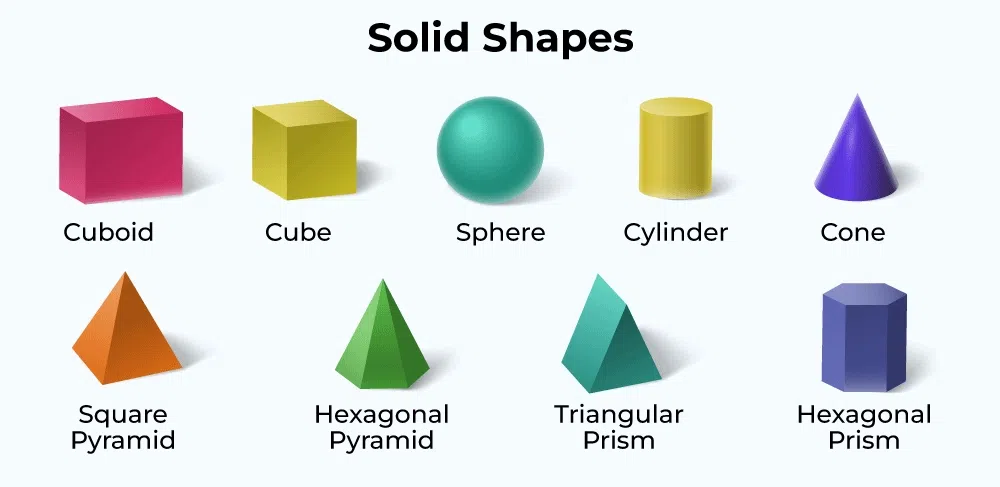Solid Geometry

### Edges

An edge is the line segment that joins one vertex to another. It helps in forming the outline of 3D shapes. It means it joins one corner point to another.

The table consists of the number of edges in different 3D shapes :

Solid Shapes

No. of. Edges

Triangular Prism

9

Cube

12

Rectangular prism

12

Pentagonal Prism

15

Hexagonal Prism

18

Triangular Pyramid

6

Square Pyramid

8

Pentagonal Pyramid

10

Hexagonal Pyramid

12

### Faces

It is defined as the flat surface enclosed by edges that geometric shapes are made up of. It is a 2D figure for all 3D figures.

The list of the number of faces in different shapes is given below:

Solid Shapes

No. of. Faces

Triangular Prism

5

Cube

6

Rectangular prism

6

Pentagonal Prism

7

Hexagonal Prism

8

Triangular Pyramid

4

Square Pyramid

5

Pentagonal Pyramid

6

Hexagonal Pyramid

7

### Vertices

A vertex is a point where the edges of the solid figure meet each other. It can be referred to as a point where the adjacent sides of a polygon meet. The vertex is the corner where edges meet.

The number of vertices in different solid shapes is given in the table:

Solid Shapes

No. of. Vertices

Triangular Prism

6

Cube

8

Rectangular prism

8

Pentagonal Prism

10

Hexagonal Prism

12

Triangular Pyramid

4

Square Pyramid

5

Pentagonal Pyramid

6

Hexagonal Pyramid

7

Examine these words in depth for various geometric forms here in the following articles:

### Three-Dimensional Geometry

Three-dimensional geometry studies the geometry of shapes in 3D space in the cartesian planes. Every point in the space is referred by 3 coordinates, (x, y,z) that are real numbers. Here is a list of articles where you can find in-depth knowledge about three-dimensional geometry.

## Solved Examples on Geometry

Example 1: If the equal angles measure 50° in an Isosceles triangle then find the third angle.

Solution:

Let the third angle be x

We know that sum of the three angles of a triangle is 180

⇒ x + 50° + 50° = 180°

⇒ x + 100° = 180°

⇒ x = 180° – 100° = 80°

Hence, the third angle measure 80°

Example 2: If one of the angle measure 70° in a parallelogram, find the rest of all the angles.

Solution:

We know that the sum of the adjacent angles of a parallelogram is 180°. Let the angle adjacent to 70° be x

⇒ 70° + x = 180°

⇒ x = 180° – 70° = 110°

We also know that opposite angles of a parallelogram are equal. Hence, the angle opposite 70° will be 70° and the angle opposite 110° will be 110°

Example 3: If a line of length 3 cm is perpendicular to the Chord of the circle 8 cm then find the radius of the circle.

Solution:

We know that a perpendicular from the center to the chord bisects the chord. Hence, the line from the center will touch the midpoint of the chord such that the length of the line on either side measures 4 cm. Now the perpendicular from the center, half of the chord, and the radius will form a right-angled triangle where the radius will be the hypotenuse of the triangle. Hence, the radius of the circle will be given by using the Pythagoras theorem,

r = √32 + 42 = √25 = 5 cm

Example 4: Find the area of the triangle whose base is 24 cm and height is 12 cm.

Solution:

Area of a triangle is given by 1/2 ⨯ base ⨯ height

Here, base = 24 cm, height = 12 cm

Hence, Area of the triangle is 1/2 ⨯ 24 ⨯ 12 = 144cm2

Example 5: Find the area and circumference of a circle whose radius is 7cm.

Solution:

Given that radius = 7 cm

Circumference of a Circle = 2πr = 2 ⨯ 22/7 ⨯ 7 = 44 cm

Area of Circle = πr2 = 22/7 ⨯ 7 ⨯ 7 = 154 cm2

## FAQs on Geometry

### 1. What is Geometry in maths?

Geometry is the branch of mathematics that deals with the shape, size, angles, and dimensions of objects in our day-to-day life.

### 2. What are the branches of Geometry?

The geometry can be divided into different parts:

• Algebraic Geometry
• Discrete Geometry
• Differential Geometry
• Euclidean Geometry
• Non Euclidean Geometry(Elliptical Geometry and Hyperbolic Geometry)
• Convex Geometry
• Topology

### 3. Why is Geometry important?

Geometry is necessary in our daily life to understand the various shapes and quantify them using area and volume.

### 4. What are the basics of Geometry?

The basics of geometry are the proper understanding of points, lines, and planes. It then helps in building all other concepts in geometry that are based on these basic concepts.

### 5. What is Euclidean Geometry?

In Euclidean geometry, we study planes and solid figures based on axioms and theorems given by Euclid.

### 6. What Is The Difference Between Euclidean and Non-Euclidean Geometry?

Euclidean Geometry is the study of the geometry of flat shapes on a plane, while non-Euclidean geometry is the study of the geometry of curved surfaces.

### 7. What are the 2 Types of Geometry?

Plane Geometry and Solid Geometry are the 2 types of Geometry. Plane Geometry is about 2D shapes while Solid Geometry is about 3D shapes.

### 8. What are the Basics of Geometry?

The Basics of Geometry are the understanding of Points, Lines, Line Segments, and the types of Geometry.